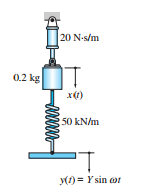### Find the expression for the steady-state response

Assignment Help Mechanical Engineering
##### Reference no: EM131285557

Find the expression for the steady-state response x(t) of the block if Y = 10 mm and ω = 600 rad/s. Does x(t) lead or lag the imposed displacement y(t)#### Determine the natural circular frequency of the disk

Determine the natural circular frequency of the disk for small oscillations by the energy method. Use the following data: W = 180 N, R = 0.6 m, k = 0.5 m (radius of gyration

#### Find the natural circular frequency of the oscillations

Determine the natural circular frequency of the oscillations for small amplitudes, assuming the motion to be simple harmonic. The mass center G is located at the distance 4R

#### Show that the differential equation of motion for the bar

The 12-kg uniform bar AOB is pinned to the carriage at O. The bar is at rest in the vertical position at t = 0 when the harmonic displacement y(t) = Y sin pt is imposed on t

#### Calculate the damping ratio

The 3-kg uniform disk oscillates about the pin at A. The pin is lubricated with heavy grease, which gives rise to rotational resistance equivalent to a viscous damping coupl

#### Write a function called sizecheck that accepts two integer

Write a function called SizeCheck that accepts two integer arguments and returns a Boolean (TRUE/FALSE). The function should return a TRUE value only if the first integer is

#### Determine the two values of the distance l

The pendulum consists of a uniform disk attached to a rod of negligible weight. Determine the two values of the distance L for which the period of the pendulum is 1.4 s for

#### Calculate the period for small oscillations

The hoop to which the pendulum is rigidly attached rolls without slip ping on the horizontal surface. Neglecting the masses of the hoop and the rod, calculate the period for

#### Determine the natural circular frequency of oscillations

The homogeneous semicircular hoop rocks back and forth on the horizontal surface without slipping. Determine the natural circular frequency of oscillations for small amplitu

### Write a Review